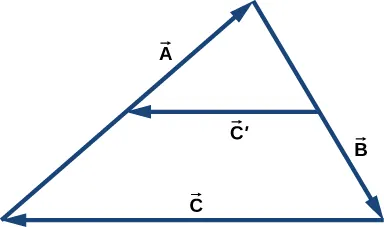University Physics Volume 1

# Challenge Problems

University Physics Volume 1Challenge Problems

### Challenge Problems

88.

Vector $B→B→$ is 5.0 cm long and vector $A→A→$ is 4.0 cm long. Find the angle between these two vectors when $|A→+B→|=3.0cm|A→+B→|=3.0cm$ and $|A→−B→|=3.0cm|A→−B→|=3.0cm$.

89.

What is the component of the force vector $G→=(3.0i^+4.0j^+10.0k^)NG→=(3.0i^+4.0j^+10.0k^)N$ along the force vector $H→=(1.0i^+4.0j^)NH→=(1.0i^+4.0j^)N$?

90.

The following figure shows a triangle formed by the three vectors $A→A→$, $B→B→$, and $C→C→$. If vector $C→′C→′$ is drawn between the midpoints of vectors $A→A→$ and $B→B→$, show that $C→′=C→/2C→′=C→/2$.91.

Distances between points in a plane do not change when a coordinate system is rotated. In other words, the magnitude of a vector is invariant under rotations of the coordinate system. Suppose a coordinate system S is rotated about its origin by angle $φφ$ to become a new coordinate system $S′S′$, as shown in the following figure. A point in a plane has coordinates (x, y) in S and coordinates $(x′,y′)(x′,y′)$ in $S′S′$.

(a) Show that, during the transformation of rotation, the coordinates in $S′S′$ are expressed in terms of the coordinates in S by the following relations:

${ x ′ = x cos φ + y sin φ y ′ = − x sin φ + y cos φ . { x ′ = x cos φ + y sin φ y ′ = − x sin φ + y cos φ .$

(b) Show that the distance of point P to the origin is invariant under rotations of the coordinate system. Here, you have to show that

$x 2 + y 2 = x ′ 2 + y ′ 2 . x 2 + y 2 = x ′ 2 + y ′ 2 .$

(c) Show that the distance between points P and Q is invariant under rotations of the coordinate system. Here, you have to show that

$( x P − x Q ) 2 + ( y P − y Q ) 2 = ( x ′ P − x ′ Q ) 2 + ( y ′ P − y ′ Q ) 2 . ( x P − x Q ) 2 + ( y P − y Q ) 2 = ( x ′ P − x ′ Q ) 2 + ( y ′ P − y ′ Q ) 2 .$Order a print copy

As an Amazon Associate we earn from qualifying purchases.